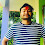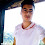# LRU Cache in Python | Let's talk about caching in Python | Tutorial on caching with functools.lru_cache

#### Need of LRU Cache while Programming

There may have been a time, when we have to run a function OVER and OVER again, let's say we are using a for loop and we have to call a function for thousands of time:

for i in range(10000):
calls_an_expensive_function()

If we could somehow, remove the cost to call that repetitive function, we will speed up our code by significant time. Here we have a very simple add function, it takes two argument "a" and "b", computes and return their sum.

print("Expensive....")
return a+b

for i in range(5):

Output

```Expensive....
69
Expensive....
69
Expensive....
69
Expensive....
69
Expensive....
69```

We are using a for loop, to call add() function multiple times with same argument. Each time we call the add() function, it recalculates the sum and return the output value even the arguments are same. For this case the calculation is simple but many times such calculation can be computationally heavy and recalculation can take a lot time.

Instead of calling the add() function every time, if we could preserve the output for known argument, our program will run faster.

#### LRU Cache in Python Standard Library

Python Standard Library provides lru_cache or Least Recently Used cache. As the name suggests, the cache is going to keep the most recent inputs/results pair by discarding the least recent/oldest entries first. Lru cache can give a significant performance boost to a function that is called repeatedly with the same arguments. If the function is called again with the same arguments, the cached value is returned, instead of calling the function again. Lru cache is how memoization can be implemented in Python.

from functools import lru_cache

@lru_cache(maxsize=2)
print("Expensive....")
return a+b

for i in range(5):

Output

```Expensive....
69
69
69
69
69```

We can see that the "Expensive..." is printed only one time, that means we are calling the function only once and it saves us a lot of computing time.

### Implementation of LRU Cache in Python

Implementing lru cache is very simple. We wrap the function with the decorators as this,

@lru_cache(maxsize=2)

lru_cache has two arguments

• maxsize
• typed

The maxsize argument says how many entries we want to consider. If maxsize=1, we will cached only 1 argument/output pair, if it is 2, we will cache 2 arguments/output pair. If maxsize is set to None, the LRU feature is disabled and the cache can grow without bound. The LRU feature performs best when maxsize is a power-of-two.

typed by default is set to False. If typed  is set to true, function arguments of different type will be cached separately. That means, sample_function(10) and sample_function(10.0) will be treated as distinct calls with distinct results.

What will happen if we set maxsize parameter to None in lru_cache?

If we set the parameter maxsize to None, the cache will grow forever for each new different argument pair. The cache size will grow in an unbounded fashion and the system will crash with a MemoryError. On the other hand, if we do not explicitly specify the maxsize parameter in lru_cache then the default value 128 will be used.

How lru_cache works in Python?

When a function wrapped with lru_cache is called, it saves the output and the arguments.
And next time when the function is called, the arguments are searched and, if the
same argument is found, the previously saved output is returned without doing
any calculation.

### Example: Using LRU Cache to print Fibonacci Series

Fibonacci Series is series of numbers in which each number is the sum of two preceding numbers. For example 1, 1, 2, 3, 5, 8 etc is a simple Fibonacci Series as 1+1 = 2, 1+2 = 3 and so on. Mathematically It can be defined asLet's use timeit to compare the time taken by the function when we use lru_cache and when we don't use the lru_cache.

import timeit
from functools import lru_cache

def fibo(n):
if n > 1:
return fib(n-1) + fib(n-2)
return n
timeit.timeit(lambda: [fibo(n) for n in range(40)], number=1)

Output
`58.56545120199917`
`It took around 59 seconds to calculate the Fibonacci series. `

Next we will wrap the function using the lru_cache decorator. Doing this, the fibonacci series will be calculated super fast. When we found the outcome of fibo(10), its output will be stored and next when we need to calculate fibo(11) the outcome of fibo(10) will be simpley added. If we don't have used the lru_cache fibo(10) need to be calculated again.

@lru_cache(maxsize=None)
def fibo_cached(n):
if n > 1:
return fib(n-1) + fib(n-2)
return n
timeit.timeit(lambda: [fibo_cached(n) for n in range(20)], number=1)

Output
`0.007842540999263292`
`When we use lru_cache it took only 0.007 seconds. `
Using the cache_info() method we can see the cache stats too. This method is used to measure the effectiveness of the cache and tune the maxsize parameter. It returns a named tuple showing hits, misses, maxsize and currsize.
fibo_cached.cache_info()
`CacheInfo(hits=0, misses=20, maxsize=None, currsize=20)`

### Example: Fetching a webpage

In this example, we will fetch a webpage using urllib,

import urllib
from functools import lru_cache

def get_example_webpage(url):
with urllib.request.urlopen(url) as response:
return html

get_example_webpage(url="https://python.org")
get_example_webpage(url="https://python.org")

This function takes url as an argument and fetch the html from particular web address.
If we run the function one time, it will take around 2 seconds and if we run the function
next it will again take around 2 seconds. Imagine we have to run the function for thousands
of time. In such case, we have to wait for very long time.
To our rescue, we got lru_cache.

@lru_cache(maxsize=1)
def get_example_webpage_cached(url):
with urllib.request.urlopen(url) as response:
return html

This function is exactly same as above but it is wrapped with lru_cache which caches the url/output pair. So if the same url is given the output will be cached. That is why when we run the function for 1st time it will take 2 seconds but when we run next, it will give output instantly. We can see the difference in the picture below.Comparison of Cached vs Not Cached

Try lru_cache on your own python interpreter and see the magic. Some tips:

• Use lru_cache when you want to reuse previously computed values.
• Do not use lru_cache to cache functions with side-effects, functions that need to create distinct mutable objects on each call.

Cheers!!

1.1.